SYLLABUS  Previous: 1.4.3 Finite elements  Up: 1.4 Numerical discretization  Next: 1.4.5 Harmonic functions

### 1.4.4 Splines

Starting from an approximation on an inhomogeneous mesh, the idea of splines is to provide a global interpolation that is continuous up to a certain derivative. For example, take a cubic Hermite spline with the 4 parameters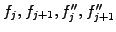, which completely determine all 4 coefficients of the cubic polynomial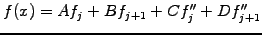(1.4.4#eq.1)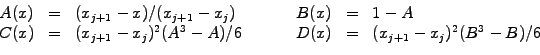It is straight forward to calculate the first and second derives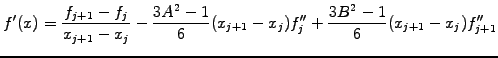(1.4.4#eq.2)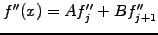(1.4.4#eq.3)

Starting with only sampled values and no information about the curvature,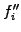are usually calculated so as to guarantee a smooth interpolation. Most common is to require that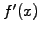be continuous from one interval to the next, leading to a tridiagonal system that holds for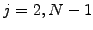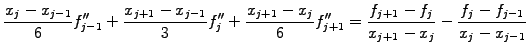(1.4.4#eq.4)

and can be solved efficiently with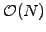operations. Two free parameters at the boundaries are used to impose the boundary conditions by choosing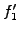and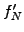(1.4.4#eq.2).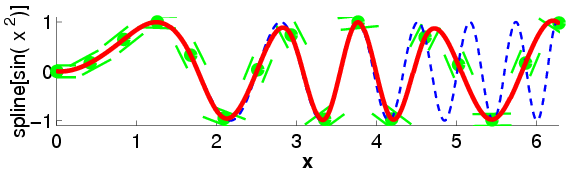Figure (1.4.4#fig.1) illustrates the procedure and shows the excellent quality of a cubic approximation until it breaks down at the limit of 2 mesh points per wavelength.

SYLLABUS  Previous: 1.4.3 Finite elements  Up: 1.4 Numerical discretization  Next: 1.4.5 Harmonic functions